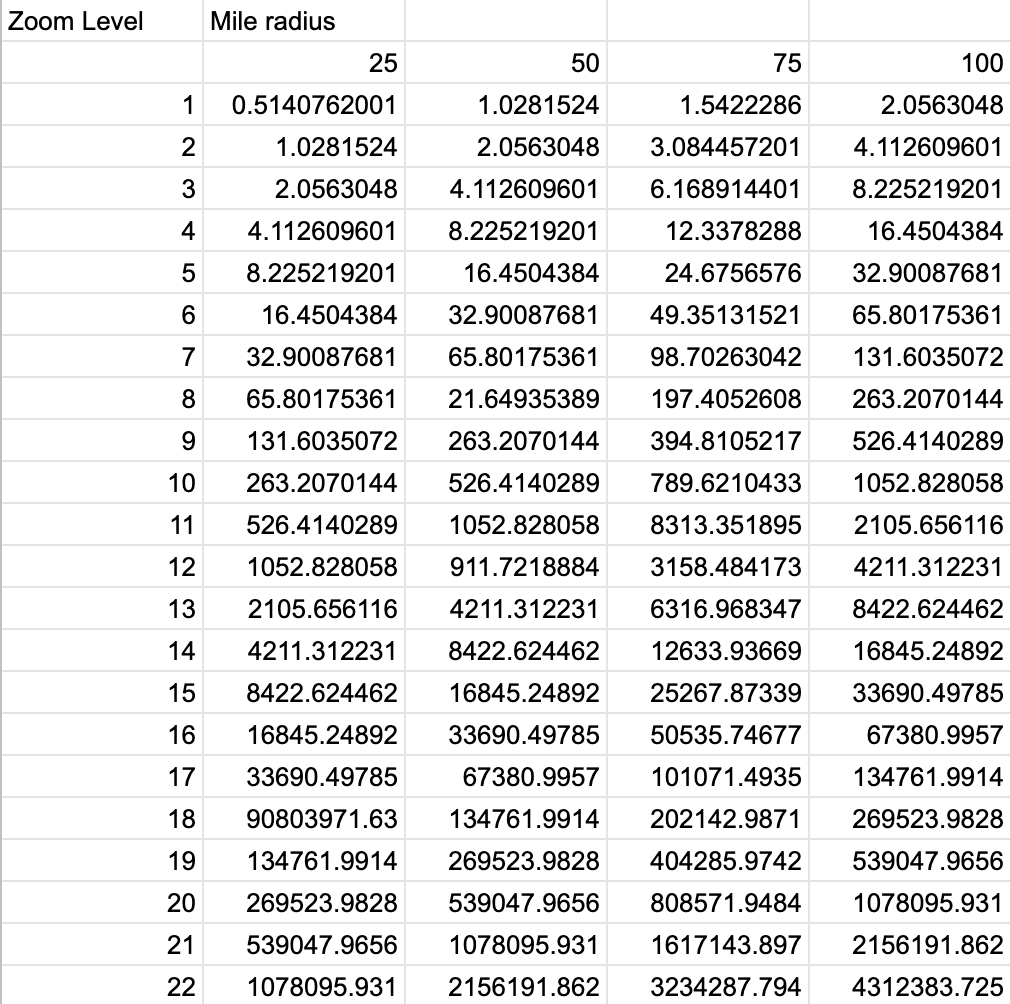# Creating Static Circles in Map Box: How many miles are in a pixel?

### Setting Up the Problem

Map Box is a live location platform that enables developers to create new worlds from location data beyond what the Google Maps API has to offer, and it is often used by developers to add a custom map to a project by generating their own layers and tile sets.

Maps are an integral part of many apps on the market today, so integrating them into your website or application has become easier in recent years. However, with the many coordinate systems and many different tools used to represent geographical data, nuances sometimes surface presenting unique challenges.

As it turns out, Mapbox doesn’t have built in capability to create a circle layer with radius measured in a distance (such as miles, kilometers, etc.); the default is pixels. My challenge was to create circles around each of the marked locations on my map tile set with radii of 25, 50, 75, and 100 miles. I also wanted the circles to remain at those relative radii as zoom level changed. While zooming out, I wanted the circles to get smaller just as the rest of the world appeared to do. The map I had currently held the circle radii at a static pixel distance:

I needed the map to be more dynamic. Since MapBox prefers the final input values to be in pixels, I had to figure out how many pixels were in 25, 50, 75, and 100 miles for each zoom level in MapBox. The zoom range in MapBox is 1-22.

### The Equation

I scoured the internet for any known relationship between zoom, pixels and distance, and eventually I came across a couple different articles with the same equation:

ground resolution = cos(latitude * π / 180) * earth circumference / map width

Knowing the circumference of the earth and the size of map tiles lets us refactor this equation to:

ground_resolution = (cos(latitude * π / 180) * 2 * π * 6378137) / (256 * 2^zoomLevel)

Or simplified:

meters/pixel = 156543.03392 * cos(latitude * π * 180) /2^ZoomLevel

There were a lot of calculations to make, and all of my data points fell between latitude 31 and 35, so I quickly computed:

cos(31 * π * 180) = 0.9999534

cos(34 * π * 180) = 0.9999431

For my purposes, I did not need to be exact, so I was comfortable with using 0.9999 as a static value in the equation in place of the cosine expression:

meters/pixel = 156543.03392 * 0.9999/2^ZoomLevel

Remember, I also needed my distances to be in miles so with a quick unit conversion and refactor I arrived at my much more easy to look at equation for pixels per mile:

pixels/mile = 2^ZoomLevel / 97.2618456

And to get pixel output:

pixels = 2^ZoomLevel * (# of miles) / 97.2618456

Finally, something I could work with. Now I could just plug in my zoom level and mile radius to return the radius in pixels.

### Generating the Map

I quickly whipped up a data table to obtain all the pixel values in range using the above derived equation:Luckily, Map Box lets you specify the rate of change for different zoom levels on your layers, so I only had to input the first (zoom level 1) and last (zoom level 22) pixel values into MapBox and set the Rate of Change to “exponential” with a base of 2 just like in our equation:Alas, I had a map with circles that were always 25, 50, 75, and 100 miles in radius no matter the zoom level. A fun little work around to get the good-looking map I desired.

### Written by Reed Van Anrooy

Software Engineer at Lotus Apps
Find me on: